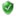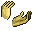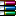Skip to main content

# Listbox Oto Sütun Genişliği#1
Visual Basic Code
``````Private Sub Form_Load()

Dim rs As DAO.Recordset
Dim intLoop As Integer
Dim strSQL As String
Dim Genislik

Me.Visible = True
DoCmd.Maximize
strSQL = "SELECT MAX(LEN(Field1)) as Len1, MAX(LEN(Field2)) as Len2, " _
& "MAX(LEN([Field3])) as Len3, MAX(LEN([Field4])) as Len4, " _
& "MAX(LEN([Field5])) as Len5, MAX(LEN([Field6])) as Len6, " _
& "MAX(LEN([Field7])) as Len7, MAX(LEN([Field8])) as Len8, " _
& "MAX(LEN([Field9])) as Len9 , MAX(LEN([Field10])) as Len10, " _
& "MAX(LEN([Field11])) as Len11, MAX(LEN([Field12])) as Len12 FROM [OgrenciListesi]"
Set rs = CurrentDb.OpenRecordset(strSQL, , dbFailOnError)

For intLoop = 0 To rs.Fields.Count - 1
'.05 value assumes 10 characters per inch for your font/fontsize
Genislik = Genislik & ";" & rs(intLoop) * 0.1 & Chr\$(34)
Next
Genislik = Mid(Genislik, 2)

Me.OgrList.ColumnWidths = Genislik

End Sub
``````
Yukarıdaki kodlarla listbox sütun genişliğini otomatik yapmak istiyorum fakat#2
örnek eklermisiniz
POWER 'un Çekirgesi :=)
ozguryasin, 23-09-2009 tarihinden beri AccessTr.neT AİLESİ üyesidir.
Access'i Profesyonel Şekilde Öğrenmek İçin https://www.accesstr.net Ailesi Yeter.Site Kurallarını sorularınızın hızlı cevaplanması için kesinlikle okuyunuz.
#3
(05/02/2019 22:45)ozguryasin Adlı Kullanıcıdan Alıntı: örnek eklermisinizServisProgramı.rar (Dosya Boyutu: 137,75 KB | İndirme Sayısı: 5)
#4
Ek olarak resimde bulunan Sol taraftaki ok işaretli yerin sebebi ne olabilir?#5
özellikler bölümünden
kayıt seçicileri
hayır
yapın düzelir. ok işareti kaybolur.
POWER 'un Çekirgesi :=)
ozguryasin, 23-09-2009 tarihinden beri AccessTr.neT AİLESİ üyesidir.
Access'i Profesyonel Şekilde Öğrenmek İçin https://www.accesstr.net Ailesi Yeter.Site Kurallarını sorularınızın hızlı cevaplanması için kesinlikle okuyunuz.
#6
Merhaba, kodda sorgu oluşturucuda Filed1, Field2 şeklinde alan tanımlaması yapılmış, ancak tablonuzda bu alanlar yok. Kodun aşağıdaki gibi değiştirilmesi gerekli.

Visual Basic Code
``````  DoCmd.Maximize
' ReSizeForm Me

Dim rs As DAO.Recordset
Dim intLoop As Integer
Dim strSQL As String
Dim Genislik

Me.Visible = True
DoCmd.Maximize
strSQL = "SELECT MAX(LEN(SıraNo)) as Len0, MAX(LEN(Tc)) as Len1, MAX(LEN([Adı- Soyadı])) as Len2, " _
& "MAX(LEN([Cep Telefonu])) as Len3, MAX(LEN([Servis Hattı])) as Len4, " _
& "MAX(LEN([Anne Adı-Soyadı])) as Len5, MAX(LEN([Anne Cep Telefonu])) as Len6, " _
& "MAX(LEN([Baba Adı Soyadı])) as Len7, MAX(LEN([Baba CepTelefon])) as Len8, " _
& "MAX(LEN([CariKodu])) as Len9 , MAX(LEN([Borç])) as Len10, " _
& "MAX(LEN([Alacak])) as Len11, MAX(LEN([Bakiye])) as Len12 FROM [OgrenciListesi]"
Set rs = CurrentDb.OpenRecordset(strSQL, , dbFailOnError)

For intLoop = 0 To rs.Fields.Count - 1

'.05 value assumes 10 characters per inch for your font/fontsize
Genislik = Genislik & ";" & rs(intLoop) * 0.1 & Chr\$(34)
Next
Genislik = Mid(Genislik, 2)

Me.OgrList.ColumnWidths = Genislik``````
"Boş Örnek Eklerim, Yapıp Verirler" demeyin, örneğinizi hazırlayın.
Komplike kod talebiniz var ise İletişim bağlantısından bize ulaşın.
Cebelleşmezsen Öğrenemezsin.

## Bir hesap oluşturun veya yorum yapmak için giriş yapın

Yorum yapmak için üye olmanız gerekiyor

ya da